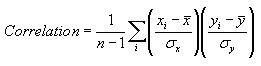Correlation

Correlation is a measure of the strength of the relationship between an investment and a benchmark.  This benchmark is commonly the S&P 500, but Fund Manager allows you to assign any investment as the benchmark.  See the Benchmark Dialog.  A benchmark must be assigned before correlation calculations can be made.

(In addition to this Custom Report field calculating the correlation relative to a benchmark investment, a Correlation Matrix Report is also available that calculates correlation of monthly returns between groups (asset type, investment goal, sector, investment type, or currency).)

The correlation coefficient can vary between -1 and 1.  A value of 1 indicates the investment and benchmark are perfectly matched.  A value of -1 indicates they are perfectly matched, but in a negative manner (when one goes up, the other will go down with the same strength).  A value of 0 indicates there is no relationship between the investment and benchmark.

#### Calculation Details:

When calculating the correlation coefficient of an investment the simple monthly returns over the specified comparison period are calculated.  The simple monthly return is:

Return = (End_price + Dist_per_share - Start_price) / Start_price

The returns for both the investment and benchmark are calculated.  If the comparison period is 5 years, there are 60 monthly returns for each the investment and the benchmark.  The correlation of these returns is calculated using the formula:where 'n' is the number of data points (60 in this example), and 'x' are the benchmark returns, and 'y' are the investment returns.  When calculating returns your current interpolation range preferences are used.  If sufficient pricing data is not available the correlation will not be reported.

The correlation coefficient for a portfolio, asset type, goal, or investment type is determined by calculating returns from a weighted average of the investments in that group.  The weighting is based on the ending value.

Fund Manager can output the data points used in this calculation to a log file.  By default the logging feature is turned off.  To turn it on, create a new registry string value at:

HKEY_CURRENT_USER\Software\Beiley Software\Fund Manager\CurrentVersion\techanal

named "benchmarklog" and set it to a value of "1".  The log file is named "stats_log.txt", and will be located in your user's AppData\Roaming\Fund Manager folder (usually a hidden folder).  This log file is deleted upon exiting Fund Manager.